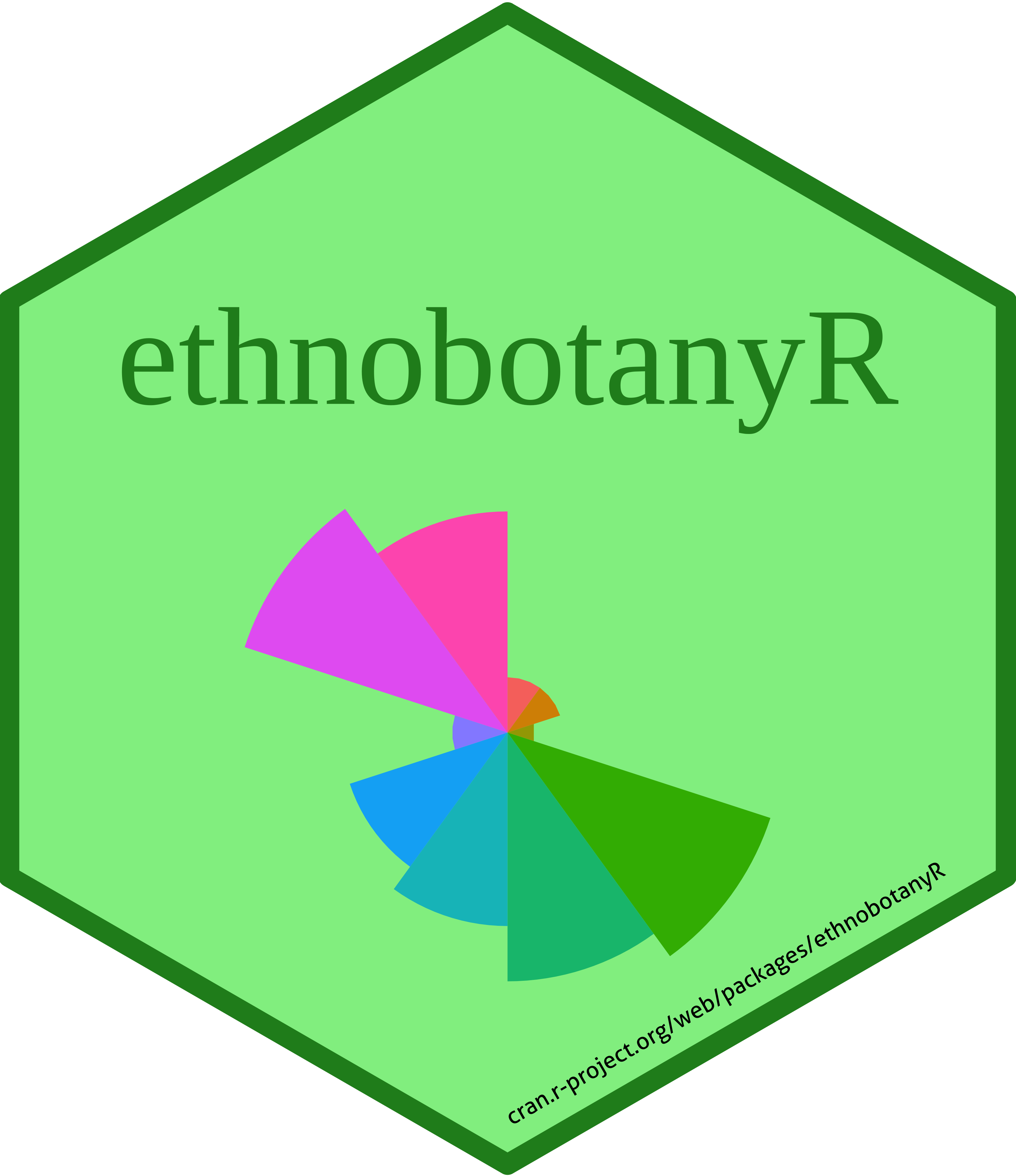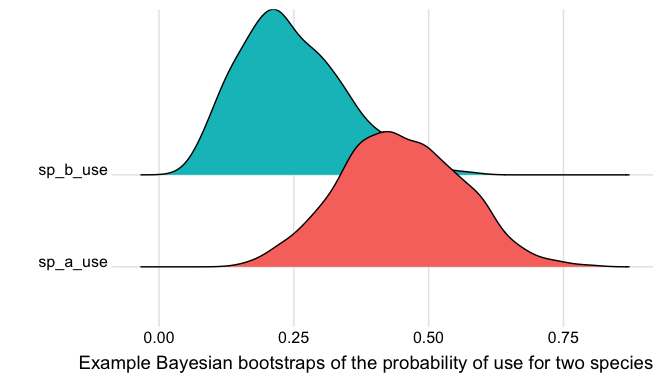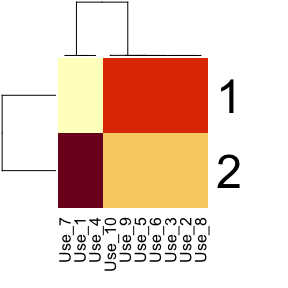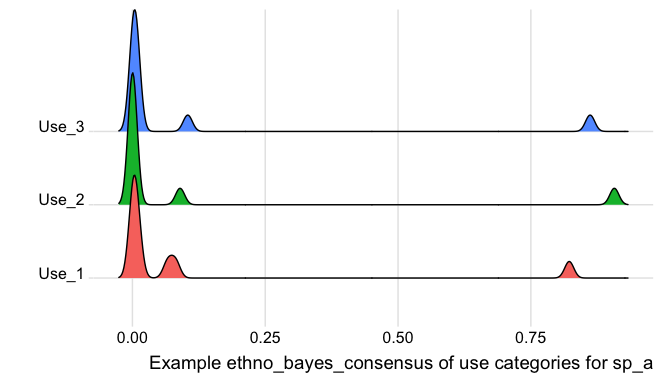# Modeling with ethnobotanyRPlease remember to cite this package if you use it in your publications.

citation("ethnobotanyR")
#>
#> To cite package 'ethnobotanyR' in publications use:
#>
#>   Whitney C (2022). _ethnobotanyR: Calculate Quantitative Ethnobotany
#>   Indices_. R package version 0.1.9,
#>   <https://CRAN.R-project.org/package=ethnobotanyR>.
#>
#> A BibTeX entry for LaTeX users is
#>
#>   @Manual{,
#>     title = {ethnobotanyR: Calculate Quantitative Ethnobotany Indices},
#>     author = {Cory Whitney},
#>     year = {2022},
#>     note = {R package version 0.1.9},
#>     url = {https://CRAN.R-project.org/package=ethnobotanyR},
#>   }

Here we will use an example data set called ethnobotanydata, which is provided to show how standard ethnobotany data should be formatted to interface with the ethnobotanyR package. This is an ethnobotany data set including one column of 20 knowledge holder identifiers informant and one of 4 species names sp_name. The rest of the columns are the identified ethnobotany use categories. The data in the use categories is populated with counts of uses per person (should be 0 or 1 values). 1

Many of the functions in ethnobotanyR make use of select() and filter_all() functions of the dplyr package (Wickham et al. 2019) and pipe functions %>% from the magrittr package (Bache and Wickham 2014). These are easy to use and understand and allow users the chance to pull the code for these functions and change anything they see fit.

First six rows of the example ethnobotany data included with ethnobotanyR
informant sp_name Use_1 Use_2 Use_3 Use_4 Use_5 Use_6 Use_7 Use_8 Use_9 Use_10
inform_a sp_a 0 0 1 0 0 0 0 1 1 0
inform_a sp_b 0 0 0 0 0 0 0 0 0 0
inform_a sp_c 0 0 0 0 0 1 1 0 0 0
inform_a sp_d 0 0 0 0 0 0 0 0 0 0
inform_b sp_a 0 1 1 0 0 1 0 0 1 0
inform_b sp_b 1 0 0 0 0 0 1 0 0 0

## ethnobotanyR modeling functions

Applying quantitative approaches in ethnobotany requires a number of preliminary steps that for a good foundation for the research. We should be clear about the aims and objectives of our work as well as the theoretical background and body of existing literature on our topic of interest. Assuming that all this is in place we can start to model systems of interest. Here we will dig in to some of the more holistic assessments and analyses that can be performed in ethnobotany.

### The non-parametric Bayesian bootstrap

Simply put, a bootstrap is any test or metric that uses random sampling with replacement, it is just one of many resampling methods. The common R function sample() is one example of a resampling method. The ethno_boot function runs a non-parametric Bayesian bootstrap, with the ability to estimate a population distribution from a set of observations, i.e. it can help us to estimate the larger population of data from which our smaller sample was derived. The procedure begins by estimating the population distribution from the data set. It then simulates the sampling process that led to the set of observations. Finally, for each sampling, the method calculates a sample statistic of interest (i.e. the mean). To calculate that sample statistic the function runs a large number of iterations, each one generating a new bootstrap replicate, and for each bootstrap replicate we calculate the sample estimate of the statistic.

Technically, the function uses the Dirichlet distribution as a way to model the randomness of a probability mass function (PMF) with unlimited options for finite sets (e.g. an unlimited amount of dice in a bag). It is the conjugate prior of the categorical distribution and multinomial distribution.

A probability mass function (PMF) is also called a frequency function, it gives probabilities for random variables that are discrete such as UR (there can be only 1 or 0 UR) or for discrete counts like plant uses where there can only be max ‘n’ people interviewed.

The Dirichlet distribution creates n positive numbers (a set of random vectors X1…Xn) that add up to 1. It is closely related to the multinomial distribution, which also requires n numbers that sum to 1.

Here we are interested in the differences (either ‘0’ no use, or ‘1’ use) between species ‘a’ and species ‘b’ or a particular use category. This could be, for example, the differences the use for a specific disease treatment between one species and another. We are using ‘Use_3’ in our data set as the specific use.

sp_a_data <- ethnobotanydata %>% filter(sp_name == "sp_a")

sp_a_use <- ethno_boot(sp_a_data$Use_3, statistic = mean, n1 = 1000) sp_b_data <- ethnobotanydata %>% filter(sp_name == "sp_b") sp_b_use <- ethno_boot(sp_b_data$Use_3, statistic = mean, n1 = 1000)

We can calculate the 90% credible interval to determine the lower bound of 0.27 and upper bound of 0.63 for species ‘a’ and 0.11 and upper bound of 0.43 for species ‘b’.

quantile(sp_a_use, c(0.05, 0.95))
#>     5%    95%
#> 0.2660 0.6281
quantile(sp_b_use, c(0.05, 0.95))
#>      5%     95%
#> 0.10600 0.43205

Running ethno_boot returns a posterior distribution of the result, i.e. it gives us an estimation, based on our observations, of what a reasonable distribution of the actual population might look like. Plotting these can give some visual probability estimation of differences between the species or informants according to the various indices.

Create a data frame and use the melt function to reshape data for the ggplot2 plotting functions.

boot_data <- data.frame(sp_a_use, sp_b_use)

ethno_boot_melt <- reshape2::melt(boot_data)
#> No id variables; using all as measure variables

Use the ggplot2 and ggridges libraries to plot the data as smooth histograms.

ggplot2::ggplot(ethno_boot_melt, aes(x = value,
y = variable, fill = variable)) +
ggridges::geom_density_ridges() +
ggridges::theme_ridges() +
theme(legend.position = "none") +
labs(y= "", x = "Example Bayesian bootstraps of the probability of use for two species")
#> Picking joint bandwidth of 0.0238The ethno_bayes_consensus function is inspired by AnthroTools package (Lane and Purzycki. 2016). It gives us a measure of the confidence we can have in the reported uses by creating a matrix of probability values. These represent the probability that informant citations for a given use are ‘correct’ (see Oravecz, Vandekerckhove, and Batchelder 2014; Romney, Weller, and Batchelder 1986).

The inputs to the function are informant responses to the use category for each plant, an estimate of informant’s with the plant, and the number of possible answers. This can be calculated with URsum or given as a value.

Depending on the size of the data this function can return a rather large set of probabilities. There are several ways to perform simple visualizations of these probabilities. Here we use the base R function heatmap (R Core Team 2019) and the the dplyr functionfilter (Wickham et al. 2019) to subset to a single species and create a ridge plot.

ethno_sp_a <- dplyr::filter(ethnobotanydata, sp_name == "sp_a")

Generate prior probabilities for all answers as a matrix. If this is not provided the function assumes a uniform distribution (prior = -1). The probability table should have the same number of columns as uses in the provided ethnobotany data and the same number of rows as there are possible answers for the consensus.

First we set the number of possible answers to ‘2’. This means informants can either agree it is ‘used’ or ‘not used’.

  answers <- 2

It is also possible to build the probability table manually using prop.table (R Core Team 2019). This can be easier if there are many answers or if there is not always a clear preference about where the higher probability should be for the various answers. This matrix must sum up to 100% chance for either ‘use’ or ‘no use’.

Here we use the dplyr function recode to reset the informant name factor variable as numeric (Wickham et al. 2019). This way we can set a prior for the informants skill for the prior_for_answers input. Assuming that informants have a varying degree of skill that we can assign as a prior for the likelihood that the data we have are correct for sp_a.

ethno_compet_sp_a <- dplyr::recode(ethno_sp_a$informant, inform_a = 0.9,inform_b = 0.5,inform_c = 0.5, inform_d = 0.9, inform_e = 0.9, inform_f = 0.5, inform_g = 0.7,inform_h = 0.5,inform_i = 0.9, inform_j= 0.9, inform_eight = 0.9,inform_five = 0.6, inform_four = 0.5,inform_nine = 0.9, inform_one = 0.5, inform_seven = 0.5, inform_six= 0.9, inform_ten = 0.9, inform_three = 0.9, inform_two = 0.5) Run the ethno_bayes_consensus function on the subset data of sp_a. ethno_sp_a_bayes <- ethnobotanyR::ethno_bayes_consensus(ethno_sp_a, answers = 2, #here we keep the default normal distribution with prior = -1 prior_for_answers = ethno_compet_sp_a)  Create a simple heatmap of the results. The heatmap function in R (R Core Team 2019) provides a good initial assessment of the results and can be a nice first look at the probability matrix that comes out of the ethno_bayes_consensus function. It includes the hclust hierarchical cluster analysis using euclidean distance for relationships among both the answers and the uses. This may be useful for looking for similarities among a number of uses or possible answers when there are more than just ‘use’ and ‘non use’ (see below). heatmap(ethno_sp_a_bayes)Here the ‘1’ and ‘2’ represent ‘use’ and ‘no use’ (y-axis). The colors are the probabilities (darker is greater). The hclust for these is not very informative since there are only 2. However, the hclust for the various uses (x-axis) might be helpful in thinking about how the strength of the information about different use categories for sp_a are grouped together. ### Richer response data Users often have a large number of counts in cells of the data set after categorization (i.e one user cites ten different ‘food’ uses but this is just one category). Let’s say that the theoretical maximum number of use reports in one category, for one species by one informant is 10. It may be useful to work with these richer datasets for the Bayes consensus analysis. The ggplot2 and ggridges libraries can be used to plot the data as smooth histograms. Here we generate some ethnobotany data with up to 10 citations in a single use category for a species by one informant. set.seed(123) #make random number reproducible ethno_sp_a_rich <- data.frame(replicate(3,sample(0:10,20,rep=TRUE))) names(ethno_sp_a_rich) <- gsub(x = names(ethno_sp_a_rich), pattern = "X", replacement = "Use_") ethno_sp_a_rich$informant <- sample(c('User_1', 'User_2'),
20, replace=TRUE)
ethno_sp_a_rich$sp_name <- sample(c('sp_a'), 20, replace=TRUE) Define the prior_for_answers of the data from these new informants in the simulated ethnobotany data. With User_1 we have high confidence because perhaps we gather this information through ‘walk in the woods’ or another method we feel good about. With User_2 we assign less confidence. Maybe did our work in a rush or gathered in another way that gives us less confidence. ethno_compet_sp_a_rich <- dplyr::recode(ethno_sp_a_rich$informant,
User_1 = 0.9, User_2 = 0.5)

We keep a normal prior for the data and the knowledge of the informants.

ethno_sp_a_bayes <- ethnobotanyR::ethno_bayes_consensus(ethno_sp_a_rich,
prior=-1) #keep a normal prior in this example with -1

Create a data frame and melt for the ggplot2 plotting functions.

ethno_sp_a_bayes_melt <-  ethno_sp_a_bayes %>%
as.data.frame() %>%
reshape2::melt()
#> No id variables; using all as measure variables

Use the ggplot2 and ggridges libraries to plot the data as smooth histograms.

ggplot2::ggplot(ethno_sp_a_bayes_melt, aes(x = value,
y = variable, fill = variable)) +
ggridges::geom_density_ridges() +
ggridges::theme_ridges() +
theme(legend.position = "none")+
labs(y= "", x = "Example ethno_bayes_consensus of use categories for sp_a")
#> Picking joint bandwidth of 0.00853Visualizing the variation in outcomes can be useful for assessing the amount of confidence we have in the cultural use of the plant across categories.

1. The example ethnobotanydata is included with the ethnobotanyR package but can also be downloaded from GitHub https://github.com/CWWhitney/ethnobotanyR/tree/master/data.↩︎